# The 9 Times Table

The 9 Times TableThe 9 times table is:

• 1 × 9 = 9
• 2 × 9 = 18.
• 3 × 9 = 27
• 4 × 9 = 36
• 5 × 9 = 45
• 6 × 9 = 54
• 7 × 9 = 63
• 8 × 9 = 72
• 9 × 9 = 81
• 10 × 9 = 90
• 11 × 9 = 99
• 12 × 9 = 108• The nine times table is made by counting up in nines.
• Apart from 11 × 9, the digits of the numbers in the nine times table add to make 9.
• To remember the nine times table up to 10 × 9 we can use a simple trick.
• Subtract one from the number to get the tens digit of the answer.
• We then think what digit needs to be added to this tens digit to make 9 and this is the units digit of the answer.
• Adding the digits of any number in the nine times table equals another number in the nine times table.

Adding up the digits of numbers in the nine times table gives us another number in the 9 times table.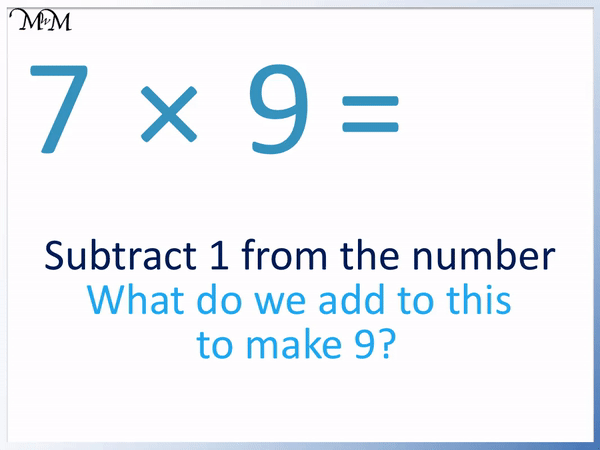• Here is 7 × 9.
• We can use a 9 times table trick to multiply numbers up to 10 by 9.
• The tens digit of our answer is one less than the number we are multiplying by 9.
• 7 – 1 = 6 and so, the answer starts with a 6.
• We then think, “What do we add to this number to make a total of 9?”
• 6 + 3 = 9 and so, the number ends in a 3.
• 7 × 9 = 63.#### 9 Times Table Flashcards

Click on the 9 times table flashcards below to memorise the 9 times table.

×
×

=

# The 9 Times Table

## What is the 9 Times Table?

The 9 times table is made by counting up in nines as shown:

• 1 × 9 = 9
• 2 × 9 = 18.
• 3 × 9 = 27
• 4 × 9 = 36
• 5 × 9 = 45
• 6 × 9 = 54
• 7 × 9 = 63
• 8 × 9 = 72
• 9 × 9 = 81
• 10 × 9 = 90
• 11 × 9 = 99
• 12 × 9 = 108

Below is the 9 times table chart.## How to Learn the 9 Times Table

To learn the 9 times table, remember that we can use a times table trick to memorise the answers up to 10 × 9. For these answers, we subtract one from the number we are multiplying by 9 to find the tens digit and the units digit is the number that we add to the tens digit to make a sum of 9.

The numbers in the 9 times table up to 12 × 9 have digits that add up to make 9. The only exception is 11 × 9 = 99, which can be remembered because we simply repeat the digit 9.

We can also remember the 9 times table because the tens digits go up by 1 with each new times table and the units digits decrease by 1 with each new times table.

The 11 × 9 = 99 times table is the only exception in which the tens digit does not change.We can see in the 9 times table chart above that we start with 0 tens and increase the digit by 1 as we go down each row. The only exception is 11 × 9 = 99, where we repeat the 9 tens digit again.

12 × 9 = 108 has 10 tens.

1 × 9 = 9 starts with 9 in the units column and then we decrease this 9 by 1 to see that 2 × 9 ends in an 8. These units digits decrease by 1 each time until we get to 10 × 9 = 90, which has 0 units.

After this, we start at 9 again in the units column with 11 × 9 = 99, which decreases by 1 again in 12 × 9 = 108.

When teaching the 9 times table, we can easily write it out by starting with 0 tens and increasing them by 1 each time and also starting with 9 units and decreasing them by 1 each time. Just remember the exception of 11 × 9 = 99, where there are still 9 tens.

We can then see that all numbers in the 9 times table from 1 × 9 = 9 up to 10 × 9 = 90 all have digits that add up to make 9.## 9 Times Table Trick

There is a trick to learn the 9 times table up to 10 × 9:

• Look at the number you are mutliplying by 9.
• Subtract one from this number to find the tens digit of your answer.
• The units digit of the answer is the digit that you add to this tens digit to make the digits of the answer add up to 9.

In general, we subtract 1 from the number and think what do we add to this answer to make 9?

For example, here is 2 × 9. The number we are multiplying is 2.

We subtract 1 from 2 to find the tens digit of the answer.

. 2 – 1 = 1 and so, the answer begins with a 1.

We think, “What do we now add to 1 to make 9?”

1 + 8 = 9 and so, the answer ends in 8.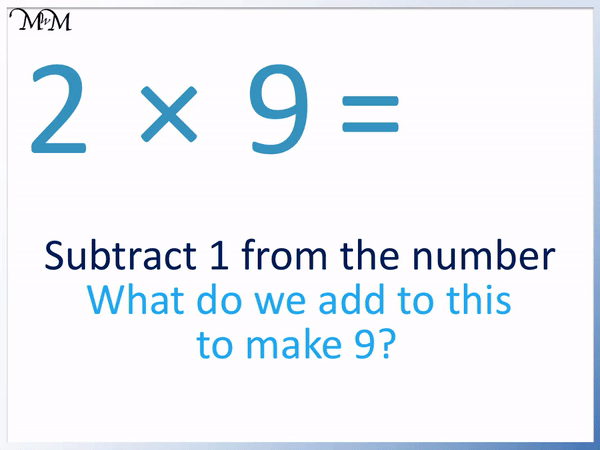2 × 9 = 18

Here is another example of using the 9 times table trick with 7 × 9.

We subtract 1 from 7 to find the tens digit of the answer.

. 7 – 1 = 6 and so, the answer begins with a 6.

We think, “What do we now add to 6 to make 9?”

6 + 3 = 9 and so, the answer ends in a 3.7 × 9 = 63

Here is 9 × 9.

We subtract 1 from 9 to find the tens digit of the answer.

. 9 – 1 = 8 and so, the answer begins with a 8.

We think, “What do we now add to 8 to make 9?”

8 + 1 = 9 and so, the answer ends in a 1.9 × 9 = 81

This times table trick works with all answers up to 10 × 9.

This means that if we know this trick, the majority of the 9 times table can be found very quickly.

This only leaves us with 2 more answers to memorise.

11 × 9 is easy because the digits in the 11 times table simply repeat. We repeat 9.

11 × 9 = 99

Finally, we can remember 12 × 9 = 108 because the digits add up to 9 again.

1 + 0 + 8 = 9

Here is the full 9 times table chart showing these last two answers.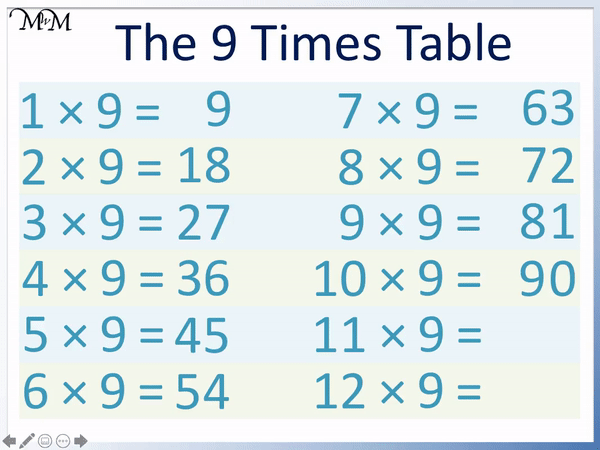Remember that we can use the pattern in the 9 times table to help remember it.

In the 9 times table chart below we can see the tens digit increase by 1 (apart from 11 × 9) and the units digit decrease by 1.

The tens digits increase as follows: 0, 1, 2, 3, 4, 5, 6, 7, 8, 9, 9, 10.

Notice the repeated 9.

The units digits decrease as follows: 9, 8, 7, 6, 5, 4, 3, 2, 1, 0, 9, 8.

Notice that once we get to 0, we start again at 9.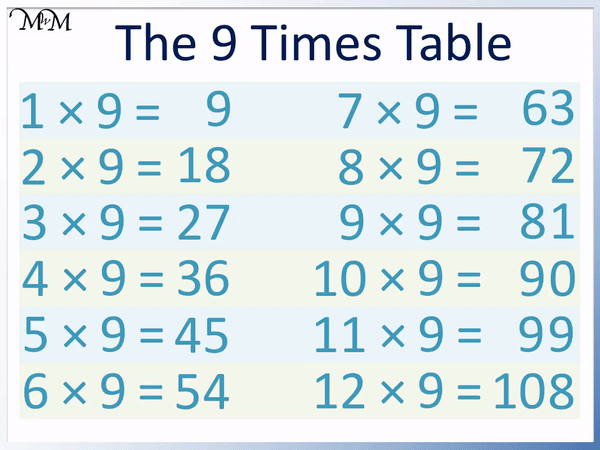Using the 9 times table trick above is the best way to learn the 9 times tables as the calculations can be done mentally.

## 9 Times Table on your Fingers

A commonly taught 9 times table trick is to use fingers.

To do the 9 times table on your fingers use the following steps:

• Hold both hands out so you can see all ten fingers.
• Count your fingers from the left and put down the finger that has the number that you are multiplying by 9.
• The number of fingers that are held up to the left of this finger is the tens digit of your answer.
• The number of fingers held up to to the right of this finger is the units digit of your answer.

This is a popular method as it allows us to see the answer to the times table without doing any calculations. As such, it can be taught as a fun way to learn a times table.

It is not as useful as the previous method of subtracting one from the number and then making the digits add up to 9. This is because this previous method allows you to do the whole process mentally and can be faster than counting fingers.Now try our lesson on Divisibility by 9 where we learn how tocheck if a number is in the 9 times table.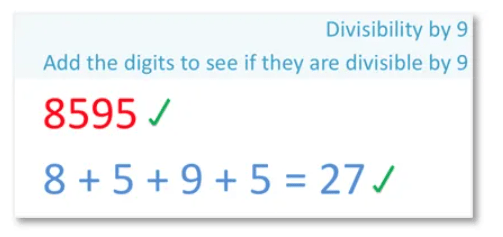error: Content is protected !!